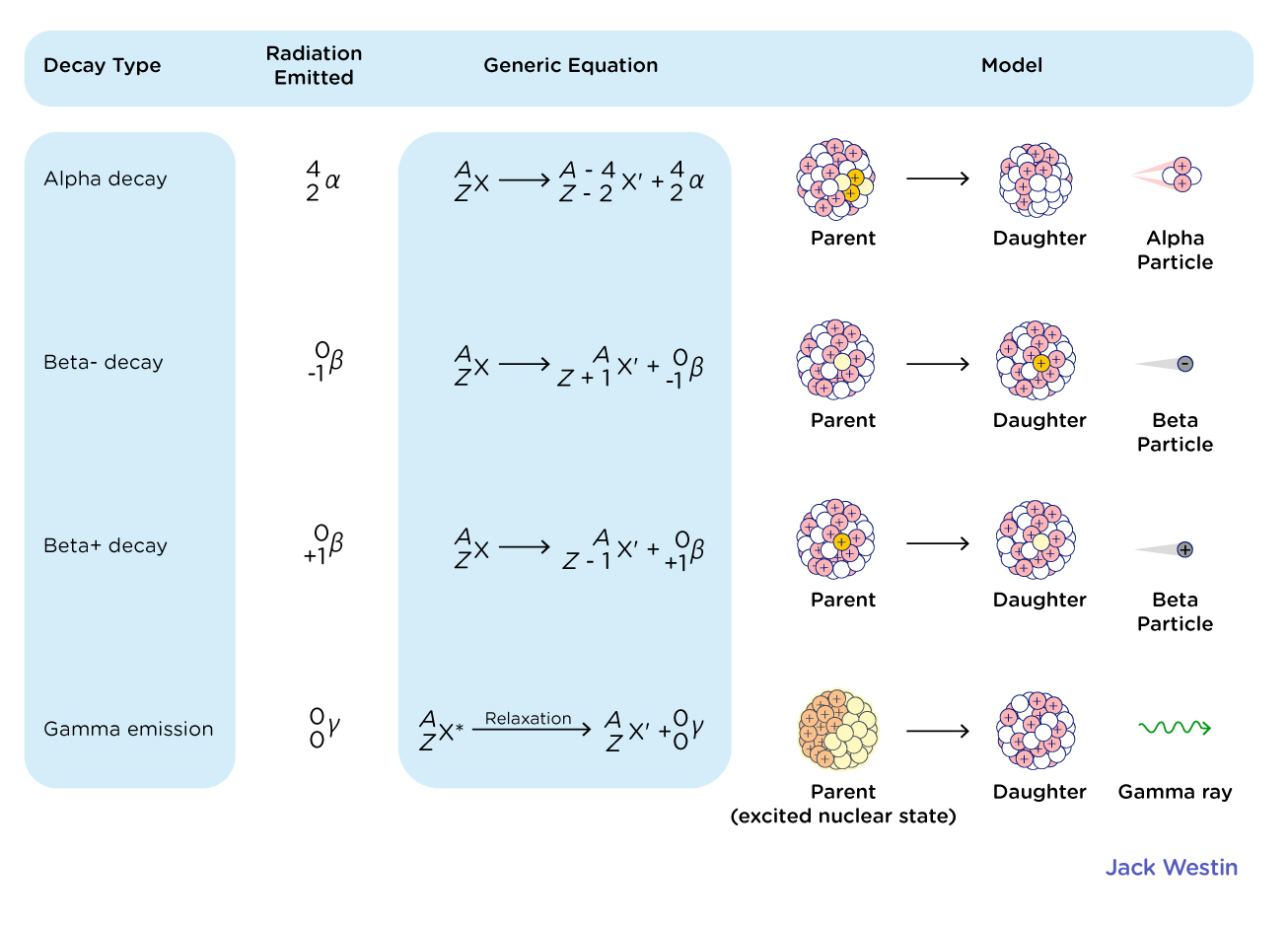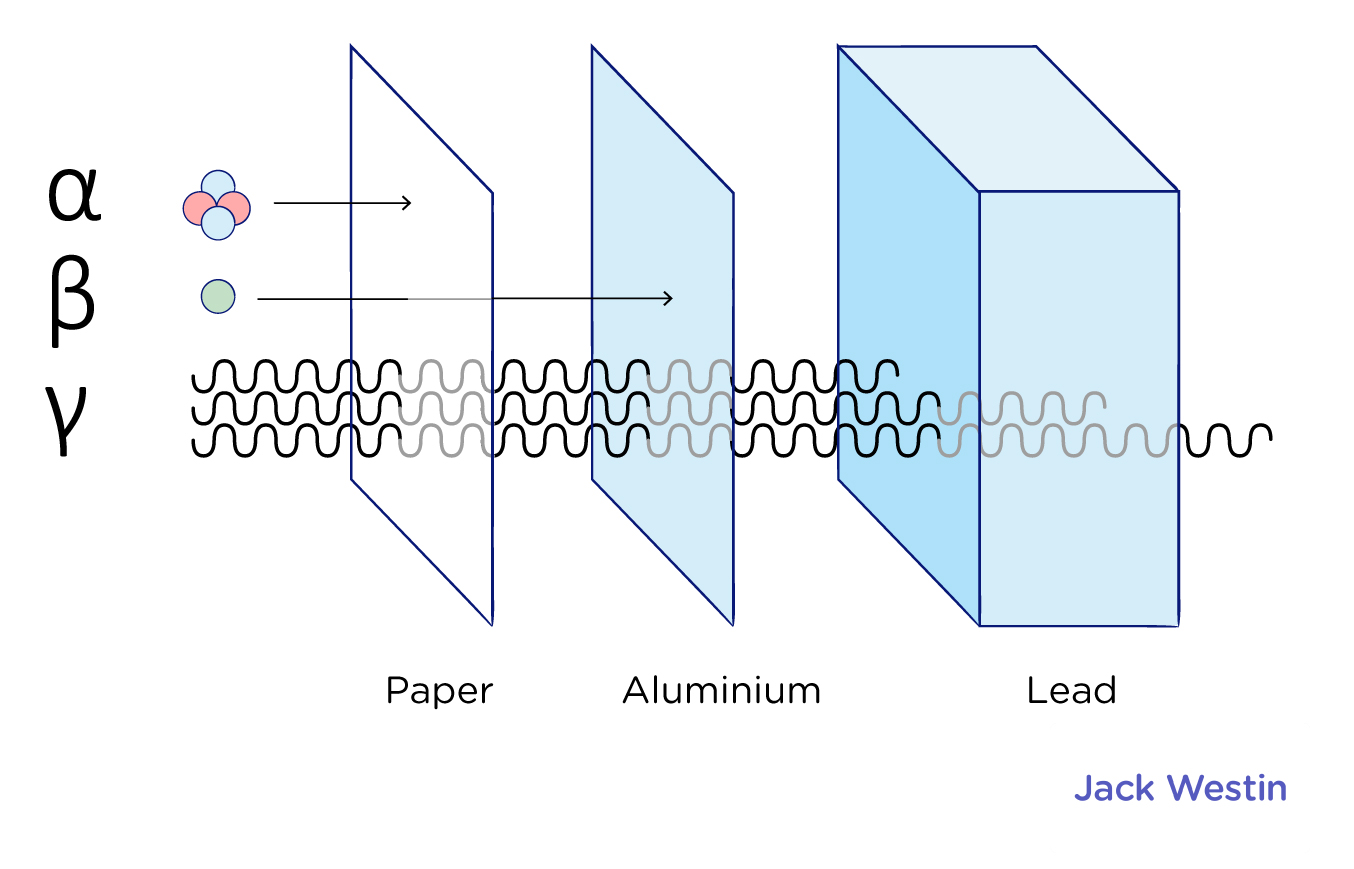Cyber Monday Sale: ALL MCAT Products & Admissions Services

MCAT Content / Atomic Nucleus / Radioactive Decay 2

Topic: Atomic Nucleus

Radioactive decay occurs when an unstable atomic nucleus emits particles or light waves.

Isotopes are atoms of the same element (thereby having the same number of protons) which differ in the number of neutrons in their nucleus. Some isotopes of a given element are more unstable than others, causing a nuclear reaction which releases energy to achieve a more stable nuclear configuration. Such isotopes are radioactive, and are referred to as “radioisotopes.”

There are many types of emitted particles and radiation that radioisotopes produce when they decay. The types we will discuss here are alpha, beta, and gamma. Alpha particles carry a positive charge, beta particles carry a negative charge, and gamma rays are neutral.Alpha particles are equivalent to a helium (He) nuclei, made of two protons and neutrons. Beta radiation is the emission a beta particle, most commonly an electron. Positrons, the antiparticle of electrons, are also beta particles and can also be emitted by beta radiation. Gamma radiation is high-energy electromagnetic waves.

Alpha decay is seen only in heavier elements greater than atomic number 52, tellurium. The other two types of decay are seen in all of the elements.The three types of radiation have different levels of penetrating power. Penetrating power refers to the energy with which the radiation particles are ejected from the atom. The higher the energy, the more the particles of light produced by radioactive decay will penetrate a substance. Alpha particles are stopped by paper, beta particles stopped by thin aluminium foil, and the most penetrating gamma is only stopped by thick lead and concrete.

To calculate the atomic properties of an atom before and after radioactive decay, two important quantities are used – the atomic mass number A (number of protons + neutrons), and the atomic number Z (number of protons). These numbers must balance before and after decay. For example, if a Uranium-238 atom (A=238, Z=92) undergoes alpha decay, then it must become Thorium-234 (A=234, Z=90) because it lost an alpha particle (He, A=4, Z=2).

Some isotopes are unstable and will undergo radioactive decay to become other elements. The radioactive decay rate is exponential and is characterized by constants, such as half-life, as well as the activity and number of particles. The half-life (t1/2) is the time taken for the activity of a given amount of a radioactive substance to decay to half of its initial value.λ, pronounced “lambda,” is the decay constant, which is the inverse of the mean lifetime (tau).

The relationship between the half-life and the decay constant shows that highly radioactive substances rapidly transform to daughter nuclides, while those that radiate weakly take longer to transform. We can measure exponential decay and the half-life of a radioactive substance using the equation below:Practice Questions

MCAT Official Prep (AAMC)

Physics Online Flashcards Question 1

Official Guide C/P Section Question 26

Physics Question Pack Passage 1 Question 1

Physics Question Pack Question 22

Physics Question Pack Passage 11 Question 68

Physics Question Pack Passage 18 Question 104

Physics Question Pack Passage 18 Question 105

Physics Question Pack Question 119

Section Bank C/P Section Passage 8 Question 60

Sample Test C/P Section Passage 10 Question 53

Sample Test C/P Section Passage 10 Question 54

Practice Exam 1 C/P Section Passage 4 Question 20

Practice Exam 1 C/P Section Passage 8 Question 42

Practice Exam 1 C/P Section Question 46

Practice Exam 2 C/P Section Question 11

Practice Exam 2 C/P Section Passage 10 Question 56

Practice Exam 4 C/P Section Passage 9 Question 49

Practice Exam 4 C/P Section Question 45

Key Points

• Radioactive decay occurs when an unstable atomic nucleus loses energy by emitting energy in the form of emitted particles or electromagnetic waves, called radiation.

• Alpha particles carry a positive charge, beta particles carry a negative charge, and gamma rays are neutral.

• An alpha particle is made up of two protons and two neutrons bound together.

• Beta particles are high energy electrons or positrons. Beta radiation occurs when a neutron turns into a proton releasing an electron.

• Gamma rays are waves of electromagnetic energy or photons.

• The atomic mass number (A) and atomic number (Z) can be used to calculate the properties of an atom after radioactive decay.

Key Terms

Isotope: a variant of a particular chemical element, which shares the same number of protons as other atoms of the element, but differs in its number of neutrons.

Alpha particle: a particle consisting of two protons and two neutrons bound together, identical to a helium nucleus.

Beta particle: a high energy electron released during beta decay.

Gamma-ray: a high-energy wave of electromagnetic energy.

Penetrating power: the energy with which the radiation particles are ejected from the atom.

Half-life: the time required for half of the nuclei in a sample of a specific isotope to undergo radioactive decay

Exponential: is a specific way that a quantity may increase over time.

Proton: Positively charged subatomic particle forming part of the nucleus of an atom and determining the atomic number of an element. It weighs one amu.

Neutron: A subatomic particle forming part of the nucleus of an atom. It has no charge. It is equal in mass to a proton, or it weighs one amu.

Electron: Each electron has a negative charge (-1) with weight so small it’s normally negligible as compared to proton or neutron.

Positron: antiparticle counterpart of an electron. Has a charge of +1.

Radioactive decay: the process by which an unstable atomic nucleus loses energy by radiation